## m个数中取n个数的组合算法

为什么80%的码农都做不了架构师？->>>1 2 3 4 5 6

1 2 3 4 5 7

1 2 3 4 5 8

1 2 3 4 5 9

......

0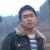#### 引用来自“Andre.Z”的答案

00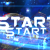m 中取n个元素等于

C(m, n) = C(m-1, n-1) + C(m-1, n)

```m = 10
n = 4
p = range(0, m)

def getC(seq, k):
if k == 0:
return [[]]
if len(seq) == k:
return [list(seq)]
res = []
for i in range(0, len(seq)-k+1):
temp = getC(seq[i:], k-1)
for t in temp:
res.append([seq[i]]+t)
return res
r = getC(p, n)
print r
```

0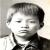0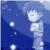```/**
* 组合问题： 输出从数组a的n个元素中选出m个元素的组合
* @param a	给定数组
* @param b	组合结果  (b中存放的是元素在a中的编号)
* @param n
* @param m
* @param M	常量=m 记录a中元素个数
*
* 思路：1.从n个元素中选出序号最大的数，然后在剩下的(n-1)个元素中选(m-1)个
* 	  当m=1时，倒序输出数组b；
*      2.从n个元素中选出编号次小的数，重复第1步。
*/
public static void comb( int[] a, int[] b, int n, int m, final int M ){
for( int i=n; i>=m; i--){
b[m-1] = i-1;
if( m>1 ){
comb(a,b,i-1,m-1,M);
}else{
for( int j=M-1; j>=0; j-- ){
System.out.print(a[b[j]]+" ");
if( j==0 )
System.out.println();
}
}
}
}```
0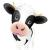n取m得到数量：

```def ngetm(n,m):
if m==n:
return 1
elif m==0:
return 1
else:
return ngetm(n-1,m-1)+ngetm(n-1,m)```

list取m得到所有结果：

```def ngetmprint(list,ans,m):
if m==len(list):
ans = ans + list
print ans
elif m==0:
print ans
else:
ngetmprint(list[1:],ans+list[0:1],m-1)
ngetmprint(list[1:],ans,m)```